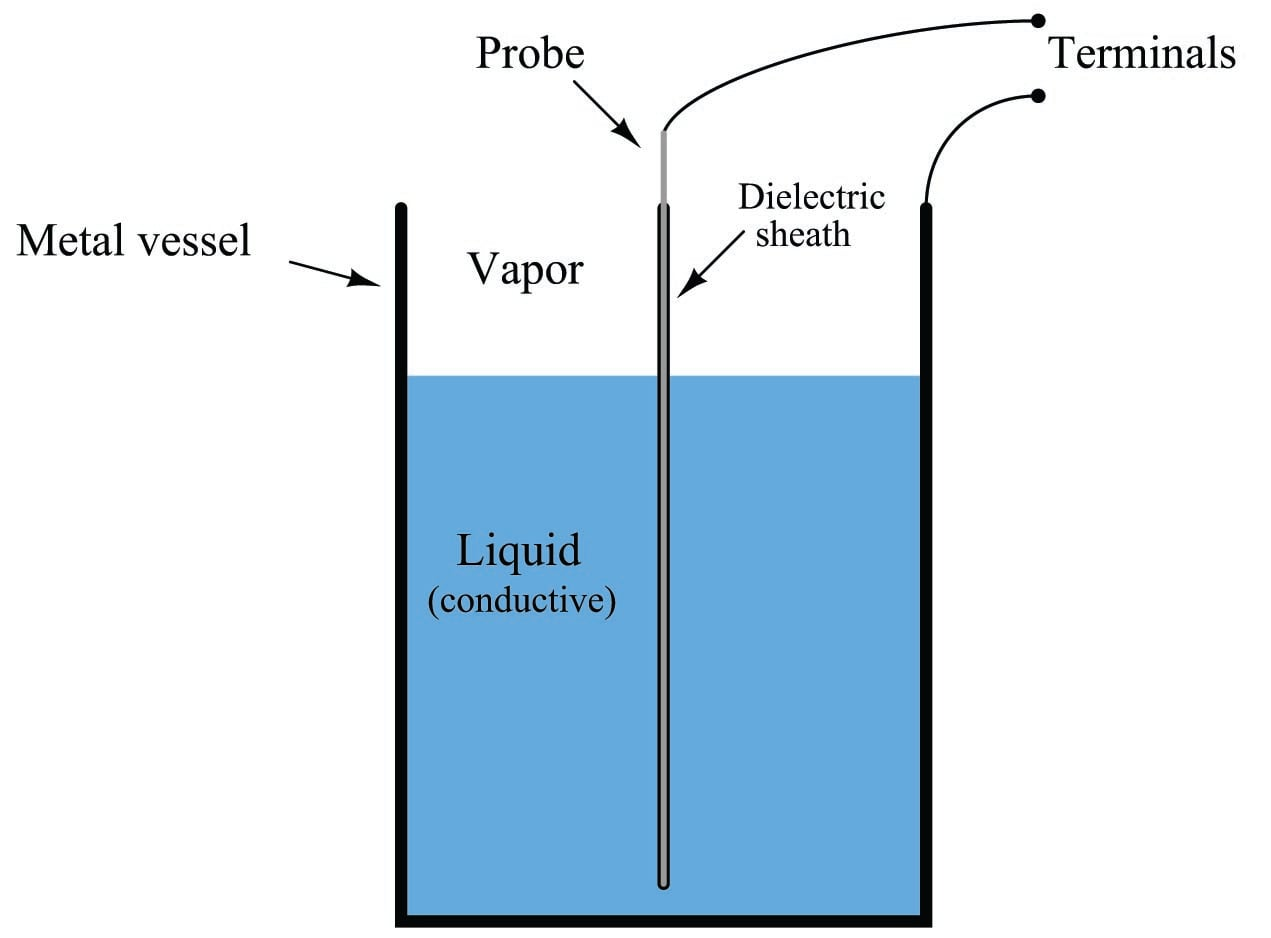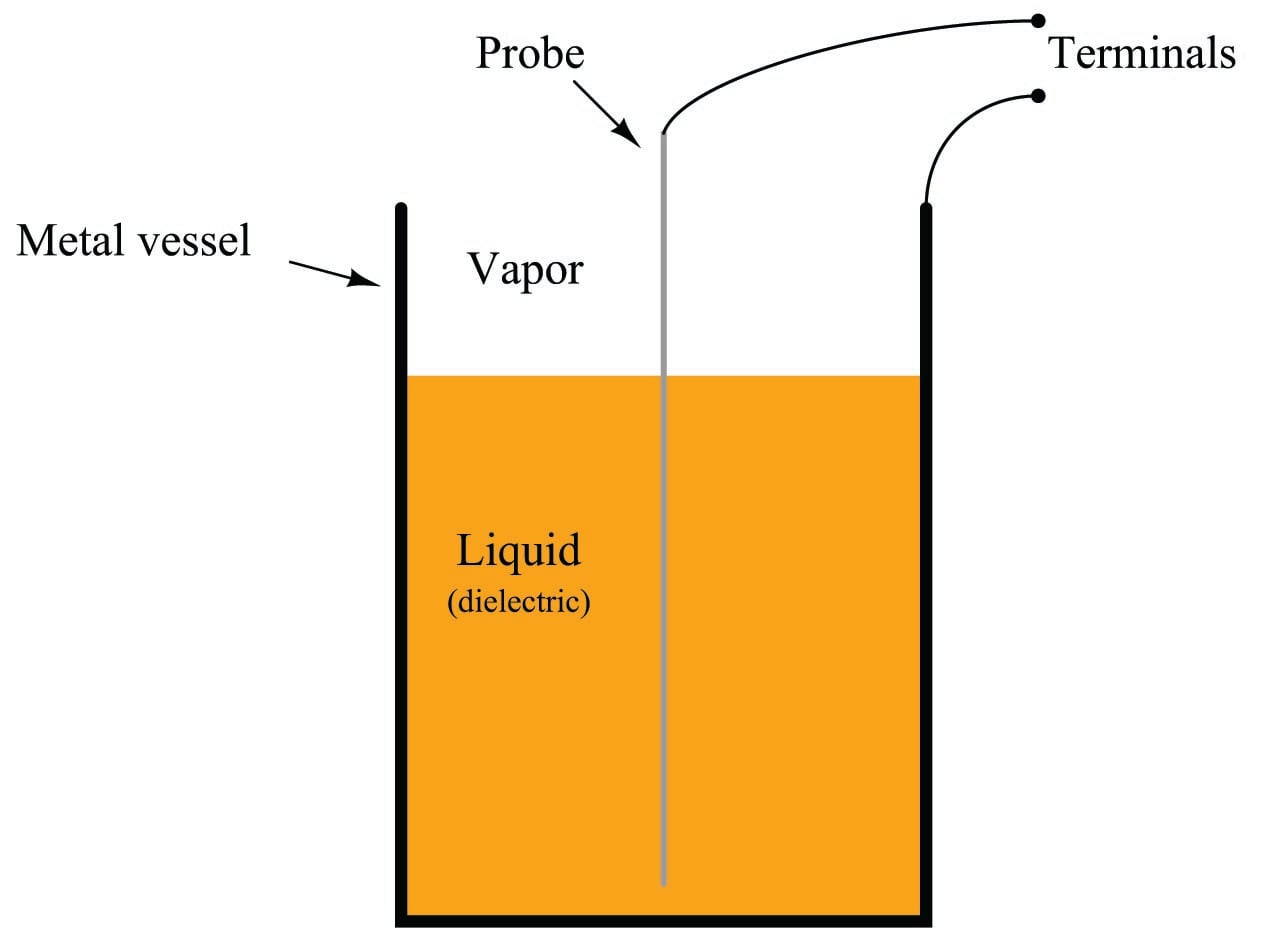Vol. Fluid Systems
Chapter Introduction to Continuous Level Measurement

# Capacitive Level Measurement

Capacitive level instruments measure electrical capacitance of a conductive rod inserted vertically into a process vessel. As process level increases, capacitance increases between the rod and the vessel walls, causing the instrument to output a greater signal.

The basic principle behind capacitive level instruments is the capacitance equation:

$C = {\epsilon A \over d}$

Where,

$$C$$ = Capacitance

$$\epsilon$$ = Permittivity of dielectric (insulating) material between plates

$$A$$ = Overlapping area of plates

$$d$$ = Distance separating plates

The amount of capacitance exhibited between a metal rod inserted into the vessel and the metal walls of that vessel will vary only with changes in permittivity ($$\epsilon$$), area ($$A$$), or distance ($$d$$). Since $$A$$ is constant (the interior surface area of the vessel is fixed, as is the area of the rod once installed), only changes in $$\epsilon$$ or $$d$$ can affect the probe’s capacitance.

Capacitive level probes come in two basic varieties: one for conductive liquids and one for non-conductive liquids. If the liquid in the vessel is conductive, it cannot be used as the dielectric (insulating) medium of a capacitor. Consequently, capacitive level probes designed for conductive liquids are coated with plastic or some other dielectric substance, so the metal probe forms one plate of the capacitor and the conductive liquid forms the other:In this style of capacitive level probe, the variables are permittivity ($$\epsilon$$) and distance ($$d$$), since a rising liquid level displaces low-permittivity gas and essentially acts to bring the vessel wall electrically closer to the probe. This means total capacitance will be greatest when the vessel is full ($$\epsilon$$ is greatest and effective distance $$d$$ is at a minimum), and least when the vessel is empty ($$\epsilon$$ of the gas is in effect, and over a much greater distance).

If the liquid is non-conductive, it may be used as the dielectric itself, with the metal wall of the storage vessel forming the second capacitor plate. The probe is just a bare metal cable or rod:In this style of capacitive level probe, the only variable affecting probe capacitance is permittivity ($$\epsilon$$), provided the liquid has a substantially greater permittivity than the vapor space above the liquid. This means total capacitance will be greatest when the vessel is full (average permittivity $$\epsilon$$ is at a maximum), and least when the vessel is empty. Distance ($$d$$) is constant with a non-conducting process liquid, being the radius of the vessel (assuming the probe is mounted in the center).

Permittivity of the process substance is a critical variable in the non-conductive style of capacitance level probe, and so good accuracy may be obtained with this kind of instrument only if the process material permittivity is accurately known. A clever way to ensure good level measurement accuracy when the material’s permittivity is not stable over time is to equip the instrument with a special compensating probe (sometimes called a composition probe) below the LRV point in the vessel that will always be submerged. Since this compensating probe is always immersed, and always experiences the same $$A$$ and $$d$$ dimensions, its capacitance is purely a function of the substance’s permittivity ($$\epsilon$$). This gives the instrument a way to continuously measure material permittivity, which it then uses to calculate the level of that material in the vessel based on the capacitance of the main probe. The inclusion of a compensating probe to measure and compensate for changes in permittivity is analogous to the inclusion of a third pressure transmitter in a hydrostatic tank expert system to continuously measure and compensate for density. It is a way to correct for changes in the one remaining system variable that is not related to changes in level.

Capacitive level instruments may be used to measure the level of solids (powders and granules) in addition to liquids. In these applications, the material in question is almost always non-conductive, and therefore the permittivity of the substance becomes a factor in measurement accuracy. This can be problematic, as moisture content variations in the solid may greatly affect permittivity, as can variations in granule size. Compensating probes may not be very useful, either, because their location (at the bottom of the vessel) may not expose them to the same degree of material granularity and moisture content experienced by the main probe.

Capacitive level instruments are generally found in applications where precision is not important. These instruments tend to suffer from errors arising from changes in process substance permittivity, changes in process vapor-space permittivity, and errors caused by stray capacitance in probe cables.

#### Lessons in Industrial Automation

Published under the terms and conditions of the Creative Commons Attribution 4.0 International Public License